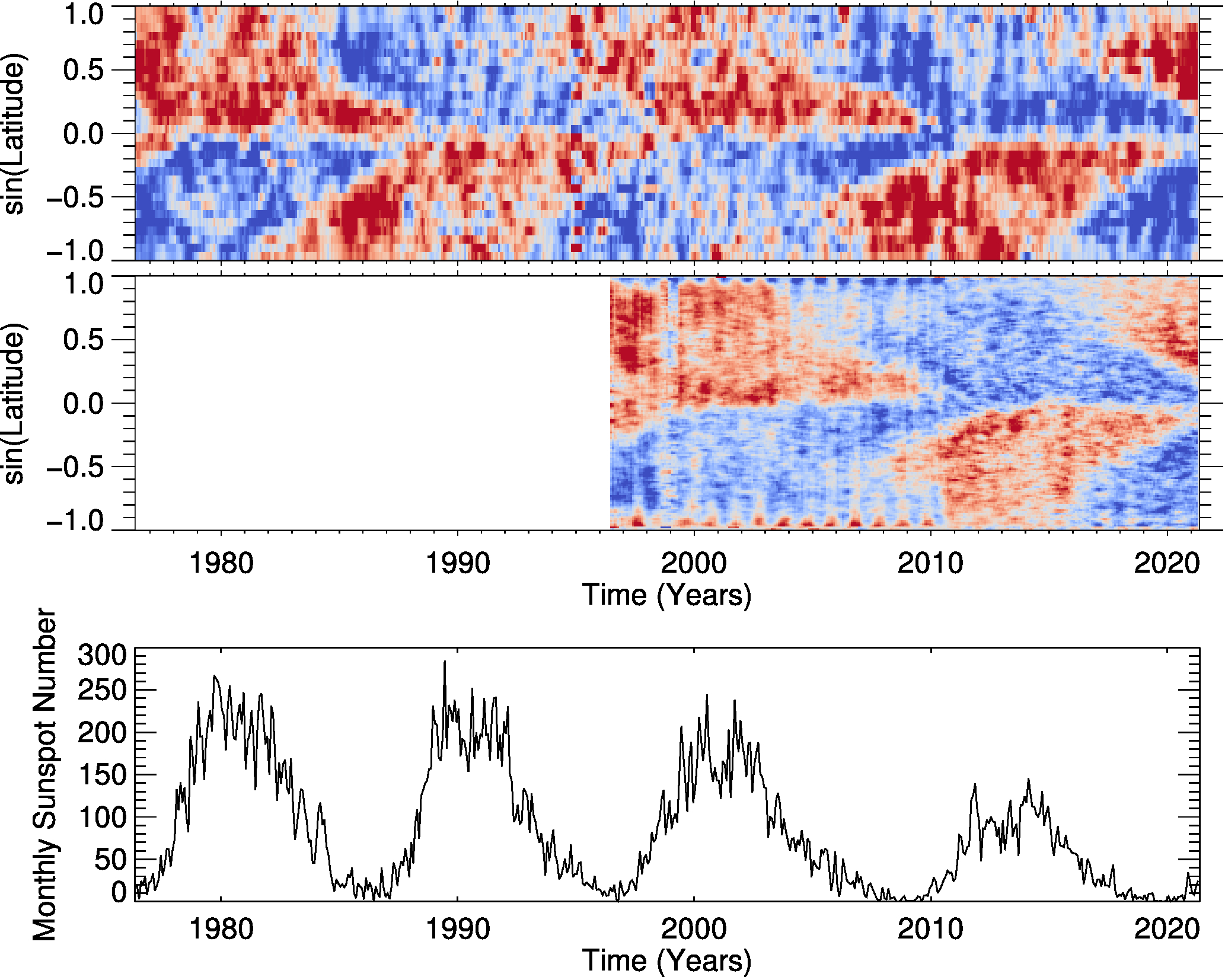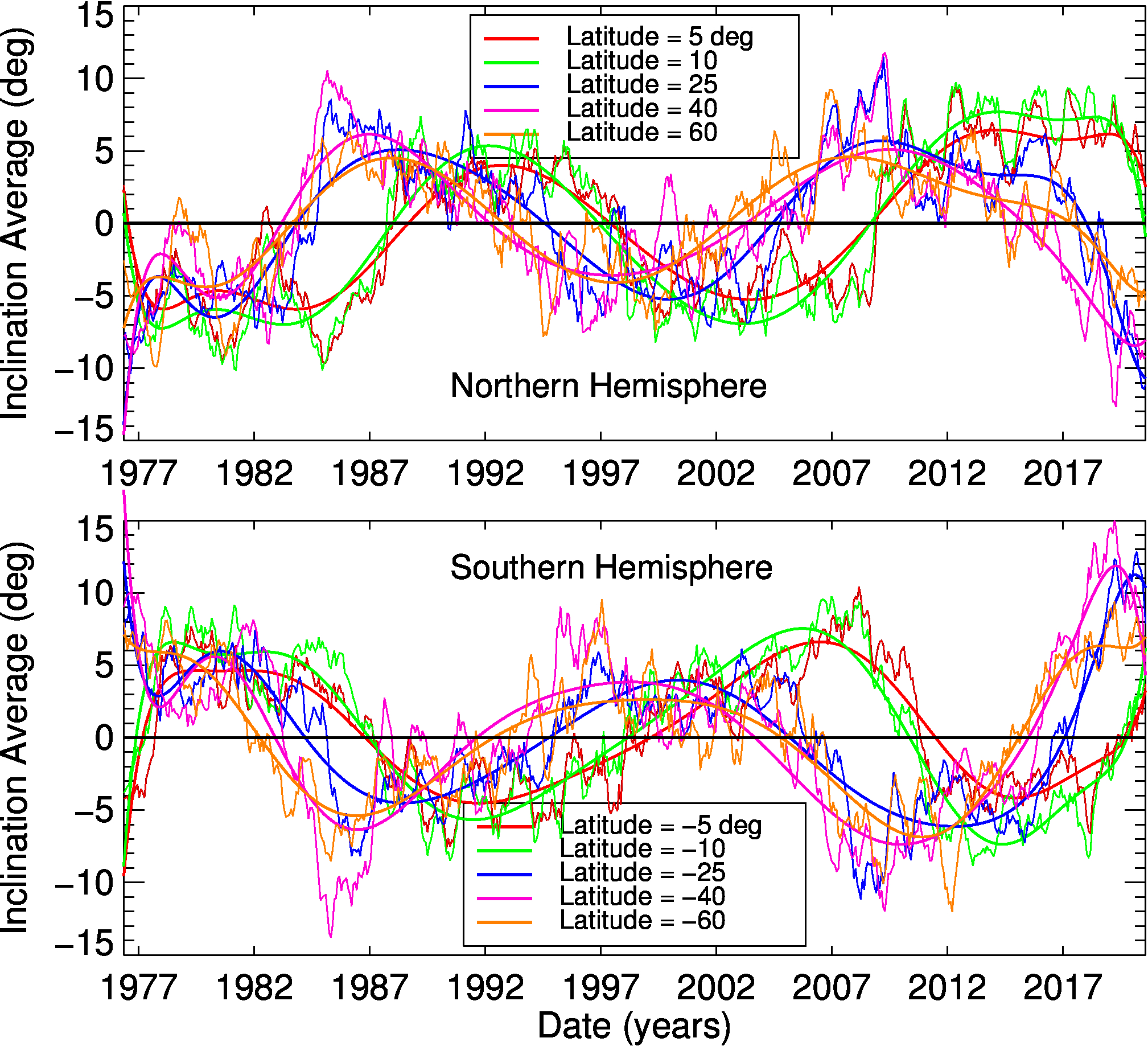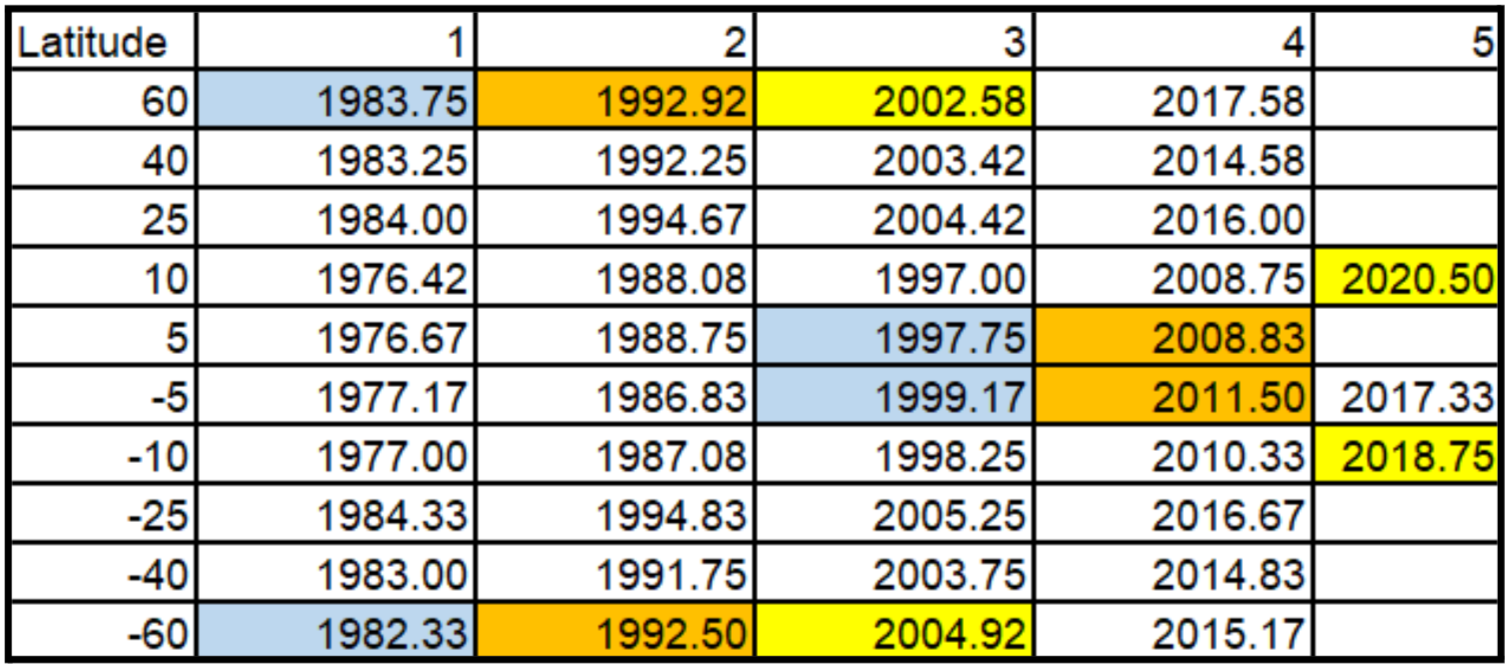# 170. Solar Toroidal Field Evolution Spanning Four Sunspot Cycles Seen By WSO, SOHO/MDI, and SDO/HMI

Contributed by Allison L. Liu. Posted on November 19, 2021

Allison L. Liu1 , P. H. Scherrer2
1. Los Gatos High School, Los Gatos, CA 95030
2. W. W. Hansen Experimental Physics Laboratory, Stanford University, Stanford, CA94305-4085

Solar toroidal field can be determined using the line-of-sight magnetic field observations. It can be used to study solar cycle evolution and detect the extended activity cycle. We derive the toroidal field from 45 years of Wilcox Solar Observatory (WSO) data, 16 years of Michelson Doppler Imager (MDI) data, and 11 years of Helioseismic and Magnetic Imager (HMI) data and study its pattern over four sunspot cycles.

The toroidal field we derive is from the east-west inclination of the magnetic field line. The inclination is the angle between the magnetic field line and the local radial vector, positive in the direction of rotation (eastward) and negative in the direction opposite to rotation (westward)1.The method we use is the same as the one used in Ref. . We track each region across the solar disk and calculated the inclination using the measured line-of-sight magnetic field that varies depending on its distance from the central meridian. The inclination is calculated separately for the positive and negative fields, where the tilt is a positive angle for tilting toward the direction of rotation, such that sunspots with negative leading and positive following spots contribute to a positive toroidal component. The toroidal field is the difference of tilts of positive and negative fields, averaged over each solar rotation. The strength of the toroidal field measured this way will be about the sine of the average 5°-tilt times the line-of-sight field at disk center. We have not attempted to do this calibration due to many sources of systematic errors and since the pattern of the changing toroidal field is itself a useful measurement. For WSO data, we use level 3 data, (a longitude, sine latitude mapping) interpolated to 30 steps in sine latitude and 5° in longitude spatial grid; for MDI 96-min cadence data, we use data from every 192 minutes and for HMI 12-min cadence data, we use data from every 3 hours, remapping the data to 1800×1440 and then averaging it down to 180×144.Figure 1| Sin(latitude)-time diagrams of toroidal fields from WSO (top) and MDI & HMI (middle). Temporal profile of sunspot number is exhibited in the bottom panel. Blue indicates a positive toroidal field with a direction of solar rotation, i.e., westward; red indicates a negative toroidal field with a direction of solar rotation, i.e., eastward.

Figure 1 shows sin(latitude)-time diagrams of the toroidal field from WSO (top) and MDI & HMI (middle), together with sunspot number with time as a reference (bottom). A sign change of the toroidal field at high latitude indicates the start of a new cycle, and a sign change at the equator implies the end of the cycle. The figure shows that the start of a new cycle occurs approximately at the maximum of a sunspot cycle and ends at the minimum of the next cycle, suggesting an extended activity cycle that is approximately 16-18 years. This supports the hypothesis in Ref.  and is consistent with previous studies)1,3,4,5.Figure 2| Temporal profiles of toroidal fields at different latitudes for the northern (top) and southern (bottom) hemispheres. The smoothed thick curves represent polynomial functions fitted to the data.

To find a more quantitative determination of the length of an extended activity cycle, we use the toroidal maps of WSO because we have enough WSO data to observe multiple activity cycles. We divide the toroidal field map into multiple horizontal bins at different latitudes, and average each latitude bin to derive the toroidal field as a function of time. We then fit each averaged data with an 11-order polynomial function. Finally, we determine the time the sign changes from the fitted function. The results are shown in Figure 2. The times of these results are shown in Table 1. Using the data in the table we calculate the time the sign changes at 5° and 60° in latitude. Subtracting the times of sign change at 60° in latitude (the start of a cycle) from the times of sign change at 5° (for the last one we use the sign change at 10°) in latitude (the end of a cycle) results in the length of the cycle. For the 6 complete sections, 3 in the northern and 3 in the southern hemispheres, the length between the sign changes at high latitude and at the equator is about 15.9 years in the northern hemisphere, and 16.6 years in the southern hemisphere. For all six samples the mean is 16.2 ± 1.9 years. This is the lower bound of the length of the extended activity cycle because the data at higher latitudes are missing.Table 1| Dates of sign change of the inclination (toroidal field) versus latitude from WSO data.

We can also fit a linear function with the points of where the sign changes at different latitudes for each extended cycle. This linear fitting method is applied to HMI data that yield much clearer results because HMI has better resolution at high latitudes than WSO. Since HMI data is shorter, we are only able to apply a linear fitting to the data for one time of sign change, starting from approximately 2015 and ending at approximately 2020. However, to determine the length of an extended solar cycle, we need data for two consecutive cycles to determine the start and end of the extended cycle. In order to find the linear fitting for the previous times of sign change, we use the linear function that we calculated for the current times of sign change and shift it parallelly to the previous times of sign change. The length between the sign change at the pole and at the equator is about 16.9 years in the northern hemisphere, and 17.7 years in the southern hemisphere. That is, the slope of the line of sign change is about the same as for WSO data.

In conclusion, we derive the toroidal field, east-west inclination of the magnetic field from magnetic field data from WSO, MDI, and HMI, utilizing methods from previous studies1. We are able to detect clear patterns of the extended activity cycle not only from WSO data that is consistent with previous studies)1,3,4, but also from both MDI and HMI data that confirm the previous findings. Using toroidal field maps derived from data from WSO and HMI, we calculate the extended activity cycle and find it to be approximately 16-18 years, which supports the results of previous studies.

### References

 Shrauner, J.A., Scherrer, P.H., 1994, Solar Phys, 153, 131
 Snodgrass, H. B., 1987, Solar Physics, 110, 35.
 Lo, L., Hoeksema, J.T., Scherrer, P.H., ASP Conf. Series, 428, 109
 Ma, William, Scherrer, P. H., 2016, HMI Nuggets, 58 (http://hmi.stanford.edu/hminuggets/?p=1657)
 R. H. Cameron, T. L. Duvall Jr., M. Schussler, H. Schunker, 2018, A&A, 609, A56